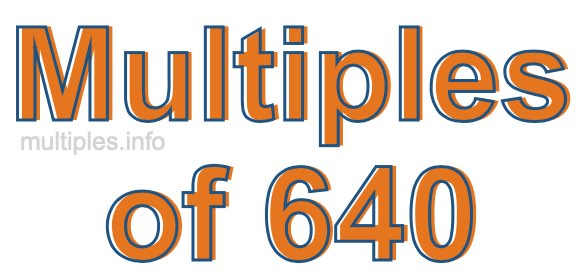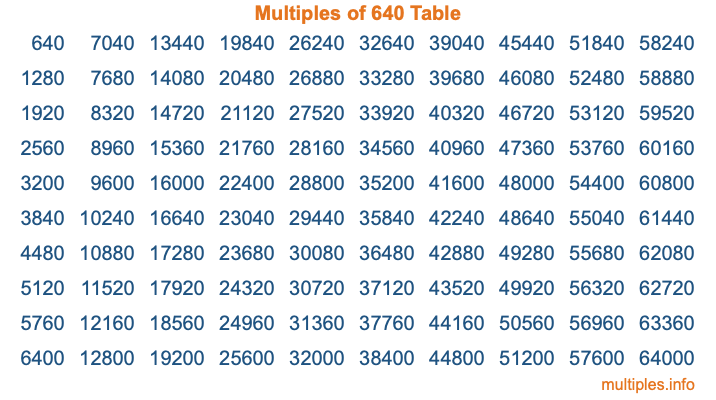Multiples of 640Welcome to the Multiples of 640 page. Here we will first teach you everything you will ever need to know about the multiples of 640, and then give you a study guide summary of everything we taught you to make sure you remember it all. Use this page to look up facts and learn information about the multiples of 640. This page will make you a multiples of six hundred forty expert!

Definition of Multiples of 640
Multiples of 640 are all the numbers that when divided by 640 equal an integer. Each of the multiples of 640 are called a multiple. A multiple of 640 is created by multiplying 640 by an integer.

Therefore, to create a list of multiples of 640, you start with 1 multiplied by 640, then 2 multiplied by 640, then 3 multiplied by 640, and so on for as long as you want. Thus, the list of the first five multiples of 640 is 640, 1280, 1920, 2560, and 3200. To see a larger list of multiples of 640, see the printable image of Multiples of 640 further down on this page. We also have a category where you can choose any nth multiple of 640.

Multiples of 640 Checker
The Multiples of 640 Checker below checks to see if any number of your choice is a multiple of 640. In other words, it checks to see if there is any number (integer) that when multiplied by 640 will equal your number. To do that, we divide your number by 640. If the the quotient is an integer, then your number is a multiple of 640.

Is  a multiple of 640?

Least Common Multiple of 640 and ...
A Least Common Multiple (LCM) is the lowest multiple that two or more numbers have in common. This is also called the smallest common multiple or lowest common multiple and is useful to know when you are adding our subtracting fractions. Enter one or more numbers below (640 is already entered) to find the LCM.

Check out our LCM Calculator if you need more details about the Least Common Multiple or if you need the LCM for different numbers for adding and subtraction fractions.

nth Multiple of 640
As we stated above, 640 is the first multiple of 640, 1280 is the second multiple of 640, 1920 is the third multiple of 640, and so on. Enter a number below to find the nth multiple of 640.

th multiple of 640

Multiples of 640 vs Factors of 640
640 is a multiple of 640 and a factor of 640, but that is where the similarities end. All postive multiples of 640 are 640 or greater than 640. All positive factors of 640 are 640 or less than 640.

Below is the beginning list of multiples of 640 and the factors of 640 so you can compare:

Multiples of 640: 640, 1280, 1920, 2560, 3200, etc.

Factors of 640: 1, 2, 4, 5, 8, 10, 16, 20, 32, 40, 64, 80, 128, 160, 320, 640

As you can see, the multiples of 640 are all the numbers that you can divide by 640 to get a whole number. The factors of 640, on the other hand, are all the whole numbers that you can multiply by another whole number to get 640.

It's also interesting to note that if a number (x) is a factor of 640, then 640 will also be a multiple of that number (x).

Multiples of 640 vs Divisors of 640
The divisors of 640 are all the integers that 640 can be divided by evenly. Below is a list of the divisors of 640.

Divisors of 640: 1, 2, 4, 5, 8, 10, 16, 20, 32, 40, 64, 80, 128, 160, 320, 640

The interesting thing to note here is that if you take any multiple of 640 and divide it by a divisor of 640, you will see that the quotient is an integer.

Multiples of 640 Table
Below is an image of the first 100 multiples of 640 in a table. The table is in chronological order, column by column. The first column has the first ten multiples of 640, the second column has the next ten multiples of 640, and so on.The Multiples of 640 Table is also referred to as the 640 Times Table or Times Table of 640. You are welcome to print out our table for your studies.

Negative Multiples of 640
Although not often discussed or needed in math, it is worth mentioning that you can make a list of negative multiples of 640 by multiplying 640 by -1, then by -2, then by -3, and so on, to get the following list of negative multiples of 640:

-640, -1280, -1920, -2560, -3200, etc.

Multiples of 640 Summary
Below is a summary of important Multiples of 640 facts that we have discussed on this page. To retain the knowledge on this page, we recommend that you read through the summary and explain to yourself or a study partner why they hold true.

There are an infinite number of multiples of 640.

A multiple of 640 divided by 640 will equal a whole number.

640 divided by a factor of 640 equals a divisor of 640.

The nth multiple of 640 is n times 640.

The largest factor of 640 is equal to the first positive multiple of 640.

640 is a multiple of every factor of 640.

640 is a multiple of 640.

A multiple of 640 divided by a divisor of 640 equals an integer.

640 divided by a divisor of 640 equals a factor of 640.

Any integer times 640 will equal a multiple of 640.

Multiples of a Number
Here you can get the multiples of another number, all with the same attention to detail as we did for multiples of 640 on this page.

Multiples of
Multiples of 641
Did you find our page about multiples of six hundred forty educational? Do you want more knowledge? Check out the multiples of the next number on our list!